# Atomic Nucleus

(redirected from Atomic Nuclei)
Also found in: Medical.

## Atomic nucleus

The central region of an atom. Atoms are composed of negatively charged electrons, positively charged protons, and electrically neutral neutrons. The protons and neutrons (collectively known as nucleons) are located in a small central region known as the nucleus. The electrons move in orbits which are large in comparison with the dimensions of the nucleus itself. Protons and neutrons possess approximately equal masses, each roughly 1840 times that of an electron. The number of nucleons in a nucleus is given by the mass number A and the number of protons by the atomic number Z. Nuclear radii r are given approximately by r = 1.2 × 10-15 m A1/3.

McGraw-Hill Concise Encyclopedia of Physics. © 2002 by The McGraw-Hill Companies, Inc.
The following article is from The Great Soviet Encyclopedia (1979). It might be outdated or ideologically biased.

## Nucleus, Atomic

the central dense part of an atom, around which the electrons revolve in quantum orbits. The mass of the nucleus is about 4 × 103 times the mass of all the electrons in the atom. The size of the nucleus is very small, 10–12–10–13 cm, which is approximately 105 times smaller than the diameter of the entire atom. The electric charge is positive and equal in absolute value to the sum of the charges of the atomic electrons (since the whole atom is electrically neutral).

The existence of the atomic nucleus was discovered by E. Rutherford in 1911 in experiments on the scattering of alpha particles upon their passage through matter. Upon observing that the alpha particles were scattered at large angles more frequently than anticipated, Rutherford hypothesized that the positive charge of the atom is concentrated in an atomic nucleus of small size (until that time, J. J. Thomson’s ideas had predominated, according to which the positive charge of the atom was assumed to be uniformly distributed throughout the volume of the atom). Rutherford’s idea was not immediately accepted by his contemporaries; the main obstacle was the belief that the electrons of the atom would inevitably fall into the nucleus because of the loss of energy to electromagnetic radiation in the course of their orbital motion about the nucleus. N. Bohr’s famous work (1913) that laid the basis for the quantum theory of the atom played a major role in the acceptance of Rutherford’s idea. Bohr postulated the stability of orbits as the initial principle of quantization of the motion of electrons in the atom, and from this principle he then deduced the regularities of optical line spectra, which explained a wealth of empirical material, such as the Balmer series. Somewhat later (late 1913), H. Moseley, Rutherford’s student, experimentally demonstrated that the displacement of the short-wave limit of the X-ray line spectra (seeX-RAY SPECTRA) of atoms as the ordinal number Z of the element in the periodic table changes corresponds to Bohr’s theory if it is assumed that the electric charge of the atomic nucleus is equal to Z (in units of electron charge). This discovery overcame the barrier of disbelief: the new physical object, the atomic nucleus, was intimately involved in a whole series of seemingly unrelated phenomena, which were now given a unified and physically obvious explanation. After Moseley’s research, the fact of the existence of the atomic nucleus was finally established in physics.

Composition of the nucleus. Up until the discovery of the atomic nucleus, only two elementary particles were known—the proton and the electron—and, accordingly, it was considered probable that the nucleus consisted of these particles (see; PROTON; and ). However, in the late 1920’s the proton-electron hypothesis encountered a serious problem, which came to be called the nitrogen catastrophe: according to the proton-electron hypothesis, the nitrogen nucleus should contain 21 particles (14 protons and seven electrons), each with spin ½; consequently, the nitrogen nucleus should have half-integral spin, but according to measurements of molecular optical spectra, the spin is equal to 1 (see and MOLECULAR SPECTRA).

The composition of the atomic nucleus was elucidated after the discovery of the neutron by J. Chadwick in 1932 (seeNEUTRON). Chadwick’s first experiments revealed that the mass of the neutron is close to that of the proton; later it was found that the spin of the neutron is equal to ½. The idea that the atomic nucleus consists of protons and neutrons was first advanced by D. D. Ivanenko (1932) and was developed soon after by W. Heisenberg (1932). The hypothesis of the proton-neutron composition of the nucleus was later completely confirmed experimentally. In modern nuclear physics, the proton (p) and neutron (n) are frequently referred to collectively as nucleons. The total number of nucleons in the atomic nucleus is called the mass number A ; the number of protons is equal to the charge of the nucleus Z (in units of electron charge), and the number of neutrons is N = AZ. Isotopes have the same Z but different A and N (seeISOTOPES), while isobaric nuclei have the same A but different Z and N.

In connection with the discovery of new particles that are heavier than nucleons, the nucleon isobars (seeRESONANCE PARTICLE), it was found that they too should be included in the makeup of the nucleus (intranuclear nucleons, upon colliding with one another, may be converted to nucleon isobars). In the deuteron, the simplest nucleus consisting of a single proton and a single neutron, the nucleons should exist as nucleon isobars about 1 percent of the time (seeDEUTERON). The existence of such isobaric states in nuclei is evidenced by a number of observed effects, especially in nuclear reactions induced by high-energy particles (see). In addition to nucleons and nucleon isobars, mesons, including pions, the lightest mesons, periodically appear in nuclei for short periods of time (10–23–10–24sec) (seeMESON and PIONS). The interaction of nucleons reduces to repeated acts of emission of a meson by one of the nucleons and absorption of the meson by another nucleon. The exchange meson currents that arise in this way particularly affect the electromagnetic properties of nuclei. The most pronounced manifestation of exchange meson currents is seen in the reaction of the splitting of a deuteron by high-energy electrons and gamma quanta.

Interaction of nucleons. The forces that bind the nucleons in the nucleus are called nuclear forces, the strongest of all interactions known in physics (seeSTRONG INTERACTION). The nuclear forces operating between two nucleons in a nucleus are of the order of a hundred times stronger than the electrostatic interaction between protons. An important property of nuclear forces is isotopic invariance, that is, they are independent of the charges of the nucleons (seeISOTOPIC INVARIANCE): nuclear interactions of two protons, two neutrons, or a neutron and a proton are the same if the particle pairs have the same states of relative motion. The magnitude of nuclear forces depends on the distance between the nucleons, the mutual orientation of the nucleon spins, the orientation of the spins with respect to the orbital moment of rotation, and the radius vector between particles. Accordingly, central, spin-spin, spin-orbital, and tensor nuclear forces are differentiated.

Nuclear forces are characterized by a certain radius of action: their potential decreases with distance r between particles faster than r–2, while the forces themselves decrease faster than r–3. An analysis of the physical nature of nuclear forces implies that they should decrease exponentially with distance. The radius of action of nuclear forces is determined by the Compton wavelength r0 of the mesons exchanged by nucleons during interaction (seeCOMPTOM WAVELENGTH):

(1) r0 = ℏ/μc

where μ is the mass of the meson, h is Planck’s constant, and c is the speed of light in a vacuum. Forces produced by the exchange of pions have the greatest radius of action. For these particles, r0 = 1.41 femtometers, or fermis (1 fm = 10–13 cm). The internu-cleon distances in nuclei are of just this order of magnitude; however, exchanges of heavier mesons (such as η-, ρ-, and ω-mesons) also make a considerable contribution to nuclear forces. It has not been precisely determined how the nuclear forces between two nucleons depend on distance, nor has the relative contribution of nuclear forces arising as a result of the exchange of different types of mesons been established with certainty. In nuclei with many nucleons there may be forces that do not reduce to interaction between only pairs of nucleons. Thus far, the part played by these many-particle forces in the structure of nuclei remains unexplained.

Size of nuclei. The size of a nucleus depends on the number of nucleons it contains. The average density ρ of the number of nucleons in the nucleus (the number per unit volume) for all many-nucleon nuclei (A > 10) is practically the same. This means that the volume of the nucleus is proportional to the nucleon number A, and the linear dimensions of the nucleus are proportional to A⅓. The effective radius R of the nucleus is defined by the relation

R = aA

where the constant a is close to but not the same as r0 and depends on the physical phenomena involved in the measurement. In the case of the nuclear charge radius, measured from the scattering of electrons by nuclei or from the position of the energy levels of μ,-mesonic atoms, a = 1.12 fm (seeMESONIC ATOM). The effective radius, determined from processes of interaction of ha-drons (nucleons, mesons, alpha particles) with nuclei, is somewhat greater than the charge radius, ranging from 1.2 to 1.4 fm.

The density of nuclear matter is fantastically great compared with the density of ordinary materials: it is equal to approximately 1014 g/cm3. In the nucleus, ρ is nearly constant in the central part and decreases exponentially toward the periphery. The following dependence of ρ on the distance r from the center of the nucleus is sometimes used for the approximate description of empirical data:

(3) ρ(r) = ρo/(1 + e(rR0)/b)

In this case, the effective radius R of the nucleus is equal to R0 + b. The quantity b characterizes the imprecision of the boundary of the nucleus and is nearly the same for all nuclei (≈0.5 fm). The parameter ρ0, which is twice the density at the “boundary” of the nucleus, is determined from the condition of normalization (the volume integral of ρ is equal to the nucleon number A). It follows from equation (2) that the size of nuclei ranges in order of magnitude from 10–13 cm to 10–12 cm for heavy nuclei (the size of the atom is about 10–8 cm). However, formula (2) describes the increase in the linear dimensions of nuclei with increasing number of nucleons only roughly, when A increases considerably. On the other hand, the change in the size of the nucleus in the case of the addition of one or two nucleons depends on the details of the structure of the nucleus and may be irregular. In particular, as shown by measurements of the isotopic shift of atomic energy levels, sometimes the radius of the nucleus even decreases with the addition of two neutrons.

Binding energy and mass of the nucleus. The binding energy ℰb is the energy that must be expended to break up the nucleus into individual nucleons. It is equal to the difference between the sum of the masses of the constituent nucleons and the mass of the nucleus multiplied by c2:

(4) ℰb = (Zmp + NMnM)c2

where mp, mn, and M are the masses of the proton, neutron, and nucleus, respectively. A remarkable feature of nuclei is the fact that ℰb is approximately proportional to the number of nucleons, so that the average binding energy per nucleon ℰb/A changes little as A changes (for most nuclei, ℰb/A ≈ 6–8 MeV). This property, called saturation of nuclear forces, indicates that each nucleon is effectively bound only to some of the nucleons in the nucleus and not to all of them (in such a case, the binding energy would be proportional to A2 for A ≫ 1). Theoretically, this is possible if the forces change sign as the distance changes (attraction at some distances is replaced by repulsion at others). Thus far, there has been no adequate explanation of the effect of saturation of nuclear forces based on the available data on the potential of interaction of two nucleons; there exist about 50 versions of the nuclear internucleon potential providing a satisfactory description of the properties of the deuteron and scattering of a nucleon by a nucleon, but not one of them can describe the effect of saturation of nuclear forces in many-nucleon nuclei.

The fact that the density p and the average binding energy per nucleon are independent of the nucleon number A creates the preconditions for the introduction of the concept of nuclear matter (an unbounded nucleus). The physical objects that correspond to the concept may be macroscopic celestial objects that have nuclear density, such as neutron stars, and in a specific sense, also ordinary nuclei with sufficiently large A.

The dependence of ℰb on A and Z for all known nuclei is approximately described by a semiempirical mass formula (first proposed by the German physicist C. F. von Weizsäcker in 1935):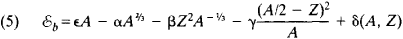Here, the first (and largest) term defines the linear dependence of ℰb on A. The second term, which reduces ℰb, is due to the fact that some of the nucleons are on the surface of the nucleus. The third term represents the energy of electrostatic (Coulomb) repulsion of protons, which is inversely proportional to the radius of the nucleus and directly proportional to the square of its charge. The fourth term accounts for the way the inequality of proton and neutron numbers in the nucleus influences the binding energy. The fifth term—δ(A, Z)—depends on the parity of the numbers A and Z and is equal to

 33.57A–¾ MeV for even A and Z (6) 0 for odd A and Z –33.57A–¾ MeV for even A and odd Z

This comparatively small correction, however, proves to be quite essential for a number of phenomena, particularly the fission of heavy nuclei. It is precisely this term that determines the fissionability of nuclei of odd-A uranium isotopes initiated by slow neutrons, which is the reason for the prominent part played by these isotopes in the production of nuclear power (see). All the constants appearing in formula (5) are chosen such that the empirical data are satisfied in the best way. Optimum agreement with experiment is given by ∊ = 14.03 MeV, α = 13.03 MeV, β = 0.5835 MeV, and γ = 77.25 MeV. Formulas (5) and (6) can be used to estimate the binding energies of nuclei that are not too far from the band of stability of nuclei. The latter is determined by the position of maximum ℰb as a function of Z at constant A. This condition determines the relation between Z and A for stable nuclei:

(7) Z = A(1.98 + 0.15A)–1

Formulas like (5) do not take into account quantum effects associated with details of nuclear structure, which can give rise to stepwise changes in ℰb close to certain values of A and Z (see below).

Structural peculiarities in the dependence of ℰb on A and Z can have a considerable effect on the question of the maximum possible value of Z, that is, on the limit of the periodic table of the elements. This limit is due to the instability of heavy nuclei with respect to the fission process. Theoretical estimates of the probability of spontaneous fission of nuclei do not rule out the possibility of “islands of stability” of superheavy nuclei close to Z = 114 and Z = 126.

Quantum characteristics of nuclei. Atomic nuclei may be in various quantum states, differing from one another in energy and other physical quantities that are conserved over time. The state with the least possible energy for a given nucleus is called the ground state, and all the other states are called excited states. The spin l and parity P are among the most important quantum characteristics of a nuclear state. The spin l is an integer for nuclei with even A and a half-integer for nuclei with odd A. The parity of the state P = ± 1 shows the change in sign of the wave function of the nucleus upon mirror reflection of space (see WAVE FUNCTION). These two characteristics are frequently combined in a single symbol, lP or l±. The following empirical rule is observed: for the ground states of nuclei with even A and Z, the spin is equal to zero and the wave function is even (lP = O+). The quantum state of a system has a certain parity P if the system has mirror symmetry, that is, if it is transformed into itself upon mirror reflection. In nuclei, mirror symmetry is somewhat violated owing to the weak interaction between nucleons (see WEAK INTERACTION), which does not conserve parity (the intensity of this interaction is of the order of magnitude of 10–5 percent of the main forces that bind nucleons in nuclei). However, the mixing of states with different parities that occurs as a result of the weak interaction is small and has virtually no effect on the structure of nuclei.

In addition to l and P, nuclear states are characterized by quantum numbers, which arise as a consequence of the dynamic symmetry of nuclear interactions (seeQUANTUM NUMBERS). The most important of these is isotopic invariance of nuclear forces. This invariance leads to the quantum number called isotopic spin, or isospin (see ISOTOPIC SPIN), in light nuclei (Z ≤ 20). The isospin T is an integer for even-A nuclei and a half-integer for odd-A nuclei. The different states of a nucleus may have different iso-spins: T ≥ (A – 2Z)/2. There is an empirical rule according to which the isospins of the ground states of nuclei are minimum, that is, equal to (A – 2Z)/2. The isospin characterizes the symmetry properties of the wave function of a given nuclear state relative to the substitution p ⇄ n. Nuclear isotopic multiplets or analog states for nuclei with the same A are associated with isospin. These states, even though they belong to different nuclei (with different Z and N), have identical structure and, consequently, identical lp and T. The number of such states is equal to 2T + 1. The deuteron, the lightest nucleus after the proton, has an isospin T = 0 and therefore has no analogs. The nuclei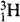andform an isotopic doublet with T = ½. In the case of heavier nuclei, the members of one isotopic multiplet are both the ground and excited states of the nuclei. This is because the Coulomb energy of the nucleus changes with Z (it increases with the number of protons); furthermore, the difference in the masses of the proton and neutron affects the total energy of the nucleus upon the substitution p ⇄ n. An example of an isotopic multiplet containing both ground and excited states is the triplet with T = 1: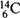(ground)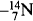(2.31 MeV)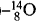(ground); the excitation energy is shown in parentheses. The half-difference of the number of neutrons and protons, called the isospin projection, is denoted by the symbol Tz. For the members of an isotopic multiplet, Tz takes on 2T + 1 values, which differ from one another by unity and lie in the interval – TTzT. The value of Tz for nuclei is defined such that Tz = –½ for a proton and Tz = + ½ for a neutron. However, in elementary particle physics, the positive value of Tz is ascribed to the proton and the negative value to the neutron. This purely arbitrary difference in definitions is dictated by conditions of convenience: with the chosen definition of Tz in nuclear physics, this quantity is positive for most nuclei.

The states of light nuclei are highly “pure” with respect to isospin—the admixtures do not exceed 0.1–1 percent in order of magnitude. Isospin is not a good quantum number for heavy nuclei (states with different isospins are mixed chiefly as a result of the electrostatic interaction of protons). Nevertheless, even in this case perceptible traces of isotopic symmetry remain; it shows up, in particular, in the presence of analog resonances (analog states that are not stable with respect to decay with the emission of nucleons).

In addition to l, P, and T, nuclear states may also be characterized by quantum numbers associated with a specific model that is used for an approximate description of the nucleus (see below).

Electric and magnetic moments of nuclei. In different states the nucleus may have magnetic dipole and electric quadrupole moments that differ in magnitude. These moments can be different from zero only when the spin l > ½. A nuclear state with definite parity P cannot have an electric dipole moment. Moreover, even in the case of nonconservation of parity, it is necessary for an electric dipole moment to arise that the interaction of nucleons be irreversible over time (T is noninvariant). Since experimental data show that T-noninvariant internucleon forces, if they exist at all, are at least 103 times weaker than the main nuclear forces and since the effects of nonconservation of parity are also very small, electric dipole moments are either equal to zero or are so small that their detection is beyond the capabilities of present nuclear experiments. Nuclear magnetic dipole moments are of the order of magnitude of the nuclear magneton. Electric quadrupole moments vary widely, from values of the order of e × 10–27 cm2(light nuclei) to e × 10–23 cm2 (heavy nuclei), where e is the electron charge. In most cases, only the magnetic and electric moments of ground states are known, since they can be measured by optical and radio-spectroscopic methods. The values of the moments depend to a large extent on the structure of the nucleus and on the distribution of the charge and currents in the nucleus. An explanation of the observed values of magnetic dipole and electric quadrupole moments is a touchstone for any theoretical model of the nucleus.

Structure and models of the nucleus. A many-particle quantum system exhibiting strong interaction (and the atomic nucleus is such a system) is an extraordinarily complex object from the theoretical standpoint. Difficulties arise not only with respect to quantitatively exact calculations of the physical quantities that characterize the nucleus but also with respect to a qualitative understanding of the basic properties of nuclear states, the spectrum of energy levels, and the mechanism of nuclear reactions. Although heavy nuclei contain many nucleons, their number is still not so great that the methods of statistical mechanics can be confidently applied, as is done in the theory of condensed media. The uncertainty of initial data on nuclear forces must also be added to the mathematical difficulties of the theory. Since internucleon interaction reduces to the exchange of mesons, in the final analysis the explanation of the properties of the nucleus must be based on the relativistic quantum theory of elementary particles, which in its present state is not without internal inconsistencies and cannot be considered complete. Although the comparatively low mean velocities of nucleons in the nucleus (0.1c) simplify the theory somewhat, making possible its construction in the first approximation on the basis of nonrelativistic quantum mechanics, the nuclear many-body problem still remains one of the fundamental problems of physics. For all these reasons, up to the present, the structure of only the simplest nuclei, the deuteron and three-nucleon nuclei 3H and 3He, has been examined ab initio. Attempts to understand the structure of more complex nuclei are based on nuclear models in which the nucleus is hypothetically likened to some simpler and better investigated physical system.

Shell model. The prototype of the shell model is the many-electron atom. According to the shell model, each nucleon in the nucleus is in a certain individual quantum state that is characterized by energy, angular momentum j and its projection m on one of the coordinate axes, and the orbital angular momentum l = j ± ½ [the parity of the state of a nucleon P = (–1)l]. The energy of the level does not depend on the projection of the angular momentum on the external axis. Therefore, in accordance with the Pauli exclusion principle, there can be (2j + 1) identical nucleons (protons and neutrons) that form a “shell” (j, l) at each energy level with momenta j, l. The total angular momentum of a filled shell is equal to zero. Therefore, if the nucleus is made up only of filled proton and neutron shells, its spin will also be equal to zero. Whenever the number of protons or neutrons reaches a magic number that corresponds to the filling of the next shell, there is a possibility of a stepwise change in certain quantities characterizing the nucleus, particularly the binding energy. This causes a kind of periodicity in the properties of nuclei depending on A and Z, analogous to the periodic law for atoms. In both cases, the physical reason for the periodicity is the Pauli exclusion principle, which forbids two identical fermions (particles with half-integral spin) from being in the same state (see PAULI EXCLUSION PRINCIPLE and FERMION). However, the shell structure in nuclei is not as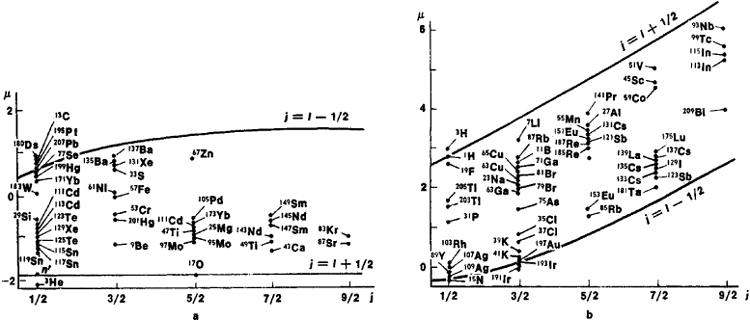Figure 1. (a) Schmidt lines for nuclei with odd number of neutrons, and (b) Schmidt lines for nuclei with odd number of protons; dots indicate experimental data

clearly delineated as in atoms. This is mainly because the individual quantum states of particles (“orbits”) in nuclei are much more strongly perturbed than in atoms; this is a result of the interaction (“collisions”) of the particles with one another. Moreover, it is known that a large number of nuclear states are nothing like an assemblage of independently moving nucleons in the nucleus; that is, they cannot be explained by the shell model. The presence of such collective states shows that the idea of individual nucleon orbits is more of a procedural basis of the theory, convenient for describing certain states of the nucleus rather than physical reality.

For this reason, the shell model has incorporated the concept of quasiparticles—elementary excitations of the medium that in many respects behave effectively like particles (seeQUASIPARTICLES). In this case, the atomic nucleus is treated as a quantum liquid, or more precisely, a Fermi liquid of finite dimensions. The nucleus in the ground state is considered as a degenerate Fermi gas of quasiparticles that effectively do not interact with each other, since any act of collision that alters the individual states of the quasi-particles is forbidden by the Pauli principle. In an excited state of the nucleus, when one or two quasi-particles are in higher individual energy levels, these particles, after leaving the orbits that they had previously occupied within the Fermi sphere, may interact both with one another and with the vacancy (hole) formed in the lower shell. As a result of interaction with an external quasiparticle, there may be a transition of quasiparticles from filled states to an unfilled state, resulting in the disappearance of the old hole and the appearance of a new one; this is equivalent to the transition of a hole from one state to another. Thus, according to the shell model, which is based on the theory of a quantum Fermi liquid, the spectrum of lower excited states of nuclei is determined by the motion of one or two quasiparticles outside the Fermi sphere and by the interaction of the quasiparticles with each other and with holes within the Fermi sphere. In this way, the explanation of the structure of a many-nucleon nucleus at low excitation energies is actually reduced to a quantum problem of two to four interacting bodies (a quasiparticle and a hole or two quasiparticles and two holes). The application of the Fermi liquid theory to atomic nuclei was developed by A. B. Migdal (1965). However, a difficulty of the theory is that the interaction of quasiparticles and holes is not weak and therefore there is no assurance that a low-energy excited state cannot occur as a result of the presence of a large number of quasiparticles outside the Fermi sphere.

Other variants of the shell model introduce effective interaction between the quasiparticles in each shell, which leads to the intermixing of the initial configurations of the individual states. Such an interaction is taken into account by the methods of perturbation theory (valid for small perturbations). The internal inconsistency of such a scheme is that the effective interaction, which is necessary for the theory to explain experimental facts, is far from weak. Moreover, a comparison of experimental and theoretical data shows that different effective interactions must be introduced in different shells, which increases the number of empirically chosen model parameters.

The principal theoretical variants of the shell model are sometimes modified by introducing different kinds of auxiliary interactions, such as the interaction of quasiparticles with oscillations of the surface of the nucleus, to achieve better agreement between theory and experiment.

Thus, the present shell model of the nucleus is actually a semi-empirical scheme that makes it possible to understand some regularities in the structure of nuclei but is not capable of providing a consistent quantitative description of the properties of the nucleus. In particular, in view of the enumerated difficulties, it is not a simple task to provide a theoretical explanation of the order of filling of the shells and consequently the “magic numbers,” which would serve as analogs of the periods in Mendeleev’s table for atoms. The order of filling of the shells depends first on the nature of the force field that determines the individual states of the quasiparticles and second on the mixing of configurations. The latter is usually taken into consideration only for unfilled shells. The experimentally observed magic numbers of neutrons (2, 8, 20, 28, 40, 50, 82, 126) and protons (2, 8, 20, 28, 50, 82) correspond to the quantum states of quasiparticles moving in a rectangular or oscillatory potential well (seePOTENTIAL WELL) with spin-orbital interaction (it is precisely because of the interaction that the numbers 28, 40, 82, and 126 arise). The explanation of the existence of magic numbers was a great achievement of the shell model, first proposed by M. G. Mayer and J. H. D. Jensen in 1949–50.

Another important result of the shell model even in its simplest form (without consideration of the interaction of quasiparticles) is that it gives the quantum numbers of the ground states of odd-mass nuclei and provides an approximate description of data on the magnetic dipole moments of such nuclei. According to the shell model, these quantities for odd-mass nuclei are determined by the state (the quantities j, l) of the last “unpaired” nucleon. In this case, l = j and P = (–1)l. The magnetic dipole moment μ (in nuclear magnetons) is equal to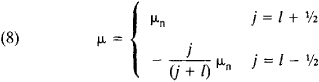if the unpaired nucleon is a neutron and toin the case of an unpaired proton. Here, μn = 1.913 and μp= 2.793 are the magnetic moments of the neutron and proton. The dependences of μ on j at a given l = j ± ½ are called Schmidt lines. According to experimental data, the magnetic dipole moments of almost all odd-mass nuclei lie between the Schmidt lines and not on the lines themselves, as required by the simplest shell model (Figure 1). Nevertheless, the experimental values of the magnetic dipole moments of nuclei are near enough to the Schmidt lines so that l can be uniquely determined in most cases if j = l and μ are known. The shell model does not work as well in describing data on the quadrupole electric moments of nuclei, both with respect to sign and to absolute value. However, it is significant that a periodicity corresponding to magic numbers is observed in the dependence of quadrupole moments on A and Z.

The diagram of the filling of nuclear shells shown in Figure 2 is based on all the foregoing information about nuclei: values of lp, electric and magnetic moments of ground states, magic numbers, and data on excited states.

Nonsphericity of nuclei; rotational model. Experimental data show extremely high values of quadrupole moments Q of nuclei with I > ½ in the region of mass numbers 150 < A < 190 and A > 200, and the values of Q differ from predictions of the shell model by a factor of 10–100. In this same region of mass numbers A, the energy dependence of lower excited states of nuclei on the spin of the nucleus shows a striking similarity to the way that the energy of a spinning top depends on its angular momentum. This is particularly pronounced for nuclei with even A and Z. In this case, the energy ℰ of an excited level with spin I is given by the relation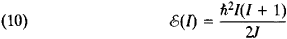where J is a quantity that is practically independent of I and has the dimensions of moment of inertia (seeMOMENT OF INERTIA). Experiments show that the spins of excited states in (10) take on only even values, 2, 4, 6, . . . (corresponding to the ground state).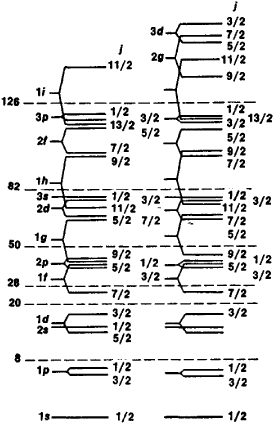Figure 2. Empirical sequence of levels of protons and neutrons in the shell model of the nucleus. The j are shown to the right of the levels, and the spectroscoplc symbol is shown to the left (the letter corresponds to a certain value of l, and the number corresponds to the level with the given l; s, p, d, f, g, h, and l denote l = 0, 1, 2, 3, 4, 5, and 6, respectively). The broken lines indicate the states that give the magic numbers when filled.

These facts are the basis for the rotational model of a non-spherical (deformed) nucleus, proposed by the American physicist J. Rainwater (1950) and developed by the Danish physicist A. Bohr and the American physicist B. Mottelson. According to the model, the nucleus is an ellipsoid of revolution. Its semimajor axis (a1 and semiminor axis (a2) are expressed in terms of the deformation parameter β of the nucleus by the relations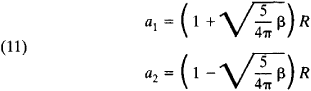The electric quadrupole moment Q of a nonspherical nucleus is expressed in terms of β. The parameters β determined from data on quadrupole moments (not only static but dynamic moments, that is, data on the probability that an excited nucleus will emit quadrupole radiation), are of the order of magnitude of 0.1 but vary fairly widely, reaching 0.5 for some nuclei of rare-earth elements. The moment of inertia of the nucleus also depends on β. A comparison of experimental data on the energy of excited states of nonspherical nuclei with formula (10) shows that the observed values of J are considerably lower than the moments of inertia of a rigid ellipsoid of revolution relative to the direction perpendicular to the axis of symmetry. Moreover, there are no rotational levels that correspond to the rotation of the ellipsoid about the axis of symmetry. These facts preclude the possibility of identifying the rotation of a nonspherical nucleus with the quantum rotation of a symmetric rigid-body top in the literal sense of the word. For the rotational model of nonspherical nuclei, a scheme is assumed that is analogous to the quantization of the motion of a diatomic molecule with identical spinless nuclei: the angular momentum of the nuclei of such a molecule relative to its center of gravity is always perpendicular to the axis of symmetry (the line joining the nuclei). Because of the properties of symmetry of the wave function with respect to the interchange of nuclei, only even values of the angular momentum (0, 2, 4, and so on) are admissible, which corresponds precisely to the values of I for rotational states of nonspherical nuclei with even A and Z. The observed values of I for nuclei with low values of β are close to the moment of inertia for the part of the ellipsoid of revolution that is outside a sphere inscribed in the ellipsoid. An ideal gas occupying a vessel in the shape of an ellipsoid of revolution could have such a moment of inertia, as could the equivalent system of particles moving independently in a nonspherical ellipsoidal potential well. As β increases, the moment of inertia of the nucleus in such a model increases fairly rapidly, reaching the rigid-body value. This contradicts experimental findings, according to which I increases much more slowly with increasing β, so that for actual nuclei the I take on values lying between the moments of inertia of the part of the ellipsoid that is outside the inscribed sphere and a rigid ellipsoid of revolution. This contradiction is eliminated by taking into account the interaction between particles moving in a potential well. It turns out that paired correlations of the “superfluid type” play a major role in this process (see below).

The described pattern of the structure of a nonspherical nucleus corresponds to a generalization of the shell model to the case of the motion of quasiparticles in a spherically asymmetric potential field (generalized model). In this connection, there is also some change in the scheme of energy levels and quantum numbers characterizing the individual orbits of the particles. With the manifestation of a physically distinct direction—the axis of symmetry of the ellipsoid—the projection of the angular momentum of each of the particles on this axis is conserved. In this case, the angular momentum j of the particle ceases to be a specific quantum number. However, for practical purposes, the mixing of orbits with different j is small for all nuclei, so that the non-sphericity of the nucleus shows up in the motion of particles mainly as an additional quantum number. For odd-mass nuclei, the spin I of the nucleus is obtained by vector addition of the angular momentum of the entire nucleus and the angular momentum of the “last” odd nucleon. In this case, the energy of the rotational level depends not only on I but also on the projection of the angular momentum K of the odd nucleon on the axis of symmetry of the nucleus. Different “rotational bands” correspond to different values of K. The general formula that defines the energy ℰ(I) of the rotational level of an odd-mass nucleus takes the form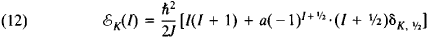where δK = 0 if K ≠ ½ and δK = 1 if K = ½; a is an empirically selected constant that characterizes the “coupling” between the angular momenta of the particle and the nucleus. The moments of inertia for even-A and odd-A nonspherical nuclei are the same in order of magnitude and are such that the excitation energy of the first rotational level for the nuclei of rare-earth elements is about 100 keV, which corresponds to values of J of about 10–47g·cm2.

An important feature of the rotational model of nonspherical nuclei is the combination of the rotation of the entire nucleus with the motion of individual nucleons in a nonspherical potential field. In this case, it is assumed that the rotation of the entire nucleus, that is, the nonspherical potential well, is fairly slow compared to the velocity of motion of the nucleons (adiabatic approximation). More precisely, this means that the distance between adjacent rotational levels should be small compared with the distances between energy levels of the nucleons in the potential well. The adiabatic approximation is not sufficient to describe the energy spectra of some nonspherical nuclei. In this case, non-adiabatic corrections are introduced (for example, for Coriolis forces), which increases the number of parameters to be determined through a comparison between theory and experiment.

A wealth of data has been obtained on the rotational spectra of nonspherical nuclei. Some nuclei are known to have several rotational bands; for example, nine bands have been observed for the 235U nucleus, and individual rotational bands have been “traced” up to spins 25/2 and beyond. Nonspherical nuclei are mainly concentrated in the region of large A. There have also been attempts to interpret some light nuclei as nonspherical; for example, the 24Mg nucleus is “suspected” of being nonspherical. The moments of inertia of such light nuclei are about ten times smaller than those of the heavy nuclei.

The rotational model of nonspherical nuclei makes it possible to describe a number of important properties of a large group of nuclei. At the same time, it is not a consistent ab initio theory; its initial assumptions have been postulated on the basis of empirical data on nuclei. The very origin of the rotational spectrum, that is, the fact that the nucleus rotates as a whole, remains unexplained in the model. Attempts to derive nuclear rotational spectra from the general quantum mechanical theory of a many-body system are as yet incomplete.

Superfluidity of nuclear matter and other nuclear models. The pairing of nucleons may give rise to superfluidity of nuclear matter, just as the pairing of electrons in metals gives rise to superconductivity (seeSUPERFLUIDITY and SUPERCONDUCTIVITY). Nucleons with momenta and spin projections of equal magnitude but opposite direction would be united into a single “particle” (Cooper pair) in an unbounded nucleus (nuclear matter). The pairing of nucleons with the same quantum numbers (j, l) and with opposite projections of the total nucleon angular momentum, equal to –j, –j + 1, . . . j – 1, j, has been hypothesized in actual nuclei. The physical cause of pairing is the interaction of particles moving in individual orbits, as assumed by the shell model. N. N. Bogoliubov was the first to note (1958) the possibility of the superfluidity of nuclear matter. One of the manifestations of superfluidity should be the existence of an energy gap between the superfluid state and the normal state of nuclear matter. The size of the gap is determined by the binding energy of the pair (the pairing energy), which should be about 1–2 MeV for nuclear matter, as nearly as can be judged from the difference in binding energies of even-mass and odd-mass nuclei. It is difficult to establish the presence of an energy gap with certainty in actual nuclei, since the spectrum of nuclear levels is discrete and the distance between shell levels is comparable to the size of the gap.

The clearest indication of the superfluidity of nuclear matter is the extent to which the moments of inertia of strongly nonspherical nuclei differ from the rigid-body values: the theory of superfluidity of nuclear matter satisfactorily explains both the absolute values of the moments of inertia and their dependence on the deformation parameter β. The theory also predicts an abrupt (stepwise) increase in the moment of inertia in a given rotational band at some critical (fairly large) spin I. This effect, which is analogous to the destruction of superconductivity by a fairly strong magnetic field, has not yet been clearly observed (there are uncertainties in the theoretical prediction of the critical values of I). The superfluidity of nuclear matter has a less pronounced but still noticeable effect on other properties of the nucleus, for example, the probabilities of electromagnetic transitions and the positions of shell levels. However, on the whole, the superfluidity of nuclear matter is less pronounced in actual nuclei than, for example, such phenomena as superconductivity of metals or superfluidity of helium at low temperatures. The reason for this is the limited size of the nucleus, which is comparable to the size of a Cooper pair. The conclusions of the theory of superfluidity of nuclei are also less reliable than those of the theory of ordinary condensed media. Here as well, the main obstacle in the theory is the fact that the interaction between nuclear particles cannot be considered weak (in contrast, for example, to the interaction that leads to electron pairing in metals). Therefore, in addition to pairwise correlations, it would be necessary to consider correlations of a greater number of particles as well (for example, four particles). Thus far, the question of the influence that such many-particle correlations have on the properties of the nucleus remains open.

The nuclear models described are the principal ones, encompassing the properties of most nuclei. However, they have been unable to describe all the observed properties of the ground and excited states of nuclei. Thus, in particular, a model of surface and quadrupole oscillations of a liquid drop that simulates a nucleus (the vibration model) is used to explain the spectrum of collective excitations of spherical nuclei. The conceptions of a cluster (block) structure of the atomic nucleus are used to explain the properties of some nuclei; for example, it is assumed that the 6Li nucleus spends a considerable part of the time as a deuteron and an alpha particle rotating about the center of gravity of the nucleus. All nuclear models play the part of more or less probable working hypotheses. However, a consistent explanation of the most important properties of nuclei firmly based on general physical principles and data on the interaction of nucleons remains as yet one of the unsolved fundamental problems of contemporary physics.

### REFERENCES

Landau, L. D., and Ia. A. Smorodinskii. Lektsii po teorii atomnogo iadra. Moscow, 1955.
Bethe, H., and F. Morrison. Elementarnaia teoriia iadra. Moscow, 1958. (Translated from English.)
Davydov, A. S. Teoriia atomnogo iadra. Moscow, 1958.
Eisenbud, L., and E. Wigner. Struktura iadra. Moscow, 1959. (Translated from English.)
Mayer, M. G., and J. H. D. Jensen. Elementarnaia teoriia iadernykh obolochek. Moscow, 1958. (Translated from English.)
Migdal, A. B. Teoriia konechnykh fermi-sistem i svoistva atomnykh iader. Moscow, 1965.
Sitenko, A. G., and V. K. Tartakovskii. Lektsii po teorii iadra. Moscow, 1972.
Rainwater, J. Uspekhi fizicheskikh nauk, 1976, vol. 120, issue 4, p. 529. (Translated from English.)
Bohr, A. Ibid., p. 545. (Translated from English.)
Mottelson, B. Ibid., p. 563. (Translated from English.)

I. S. SHAPIRO

## atomic nucleus

[ə′täm·ik ′nü·klē·əs]
(astronomy)
McGraw-Hill Dictionary of Scientific & Technical Terms, 6E, Copyright © 2003 by The McGraw-Hill Companies, Inc.
References in periodicals archive ?
of Munich, Germany) explains elementary particles, specifically the discoveries of scientists concerning electrons and atomic nuclei, the quantum properties of atoms and particles, quarks, particle accelerators, quantum electrodynamics and chromodynamics, mesons and baryons, electroweak interactions, and grand unification.
The electrons from neutron decay can also create external bremsstrahlung by collisions with atomic nuclei which is forwarded directed.
What remains is either a neutron star, a cinder of atomic nuclei so dense that one teaspoonful weighs a billion tons, or a black hole - a knot of concentrated gravity from which not even light can escape.
The subjects of bio-engineered interfaces in medicines, interstellar dust, DNA computation, conducting polymers, and the surfaces of atomic nuclei are all updated.
It is called quark-gluon plasma and they believe it is the key to understanding both the dawn of the universe and how atomic nuclei work.
This garbage is radioactive (emits deadly high-energy rays); it's generated by the nation's 104 nuclear power stations, which harness energy from the breakdown of atomic nuclei to create electricity.
In the light of Rutherford's nuclear atom (see 1911), the positive rays appeared to be streams of atomic nuclei.
It has long been observed that sub-atomic particles like electrons and atomic nuclei accelerate to very high speeds in space.
Many of the universe's heaviest elements form primarily through the r-process, a chain of reactions through which atomic nuclei climb the periodic table, swallowing up neutrons and decaying radioactively.
Starting, or initiating, the spin-based qubits then requires all the atomic nuclei to spin in the same direction, either 'up' or 'down' (clockwise or counterclockwise).

Site: Follow: Share:
Open / Close